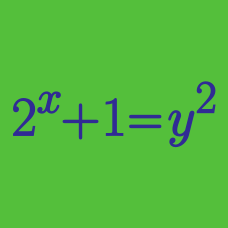Algebra

# Algebraic Manipulation Problem Solving

If $a$ and $b$ are real numbers such that $a^2+b^2=8, ab=3,$ what is the value of $\frac{a^7-ab^6-ba^6+b^7}{a+b}?$

Suppose that $x$ and $y$ are positive real numbers satisfying $x^2+y^2 = 5xy$. Then $\frac{x-y}{x+y}$ can be written as $\sqrt{\frac{a}{b}}$, where $a$ and $b$ are coprime positive integers. Find $a+b$.

$a$, $b$ and $c$ are real numbers such that $a+b+c \neq 0, a^3+b^3+c^3=12, abc=4.$ What is the value of $(a+b)(b+c)(c+a)?$

If $A-B=-4$ and $AB=62$, what is the value of $A^2+B^2$?

Given $a+b+c=4$, $a^2+b^2+c^2=24$ and $a^3+b^3+c^3=6,$ what is the value of $ab(a+b)+bc(b+c)+ca(c+a)?$

×